SBAS844 May   2017

PRODUCTION DATA.

1. Features
2. Applications
3. Description
4. Revision History
5. Device Family Comparison Table
6. Pin Configuration and Functions
7. Specifications
8. Parameter Measurement Information
9. Detailed Description
1. 9.1 Overview
2. 9.2 Functional Block Diagram
3. 9.3 Feature Description
2. 9.3.2  Clock Input
3. 9.3.3  SYSREF Input
4. 9.3.4  DDC Block
5. 9.3.5  NCO Switching
6. 9.3.6  SerDes Transmitter Interface
7. 9.3.7  Eye Diagrams
8. 9.3.8  Alarm Outputs: Power Detectors for AGC Support
9. 9.3.9  Power-Down Mode
4. 9.4 Device Functional Modes
5. 9.5 Register Maps
10. 10Application and Implementation
1. 10.1 Application Information
1. 10.1.1 Start-Up Sequence
2. 10.1.2 Hardware Reset
3. 10.1.3 SNR and Clock Jitter
4. 10.1.4 Power Consumption in Different Modes
5. 10.1.5 Using DC Coupling in the ADC32RF42
1. 10.1.5.1 Bypassing the Offset Corrector Block
2. 10.2 Typical Application
11. 11Power Supply Recommendations
12. 12Layout
13. 13Device and Documentation Support
14. 14Mechanical, Packaging, and Orderable Information

• RMP|72

## 7 Specifications

### 7.1 Absolute Maximum Ratings

over operating free-air temperature range (unless otherwise noted)(1)
MIN MAX UNIT
Supply voltage range AVDD19 –0.3 2.1 V
AVDD –0.3 1.4
DVDD –0.3 1.4
Voltage applied to input pins INAP, INAM and INBP, INBM –0.3 AVDD19 + 0.3 V
CLKINP, CLKINM –0.3 AVDD + 0.6
SYSREFP, SYSREFM, SYNCBP, SYNCBM –0.3 AVDD + 0.6
SCLK, SEN, SDIN, RESET, PDN, GPIO1, GPIO2, GPIO3, GPIO4 –0.2 AVDD19 + 0.2
Voltage applied to output pins –0.3 2.2 V
Temperature Operating free-air, TA –40 85 °C
Storage, Tstg –65 150
Stresses beyond those listed under Absolute Maximum Ratings may cause permanent damage to the device. These are stress ratings only, which do not imply functional operation of the device at these or any other conditions beyond those indicated under Recommended Operating Conditions. Exposure to absolute-maximum-rated conditions for extended periods may affect device reliability.

### 7.2 ESD Ratings

VALUE UNIT
V(ESD) Electrostatic discharge Human-body model (HBM), per ANSI/ESDA/JEDEC JS-001(1) ±1000 V
Charged-device model (CDM), per JEDEC specification JESD22-C101(2) ±500
JEDEC document JEP155 states that 500-V HBM allows safe manufacturing with a standard ESD control process.
JEDEC document JEP157 states that 250-V CDM allows safe manufacturing with a standard ESD control process.

### 7.3 Recommended Operating Conditions

over operating free-air temperature range (unless otherwise noted)
MIN NOM MAX UNIT
Supply voltage(2) AVDD19 1.8 1.9 2.0 V
AVDD 1.1 1.15 1.25
DVDD 1.1 1.15 1.2
Temperature Operating free-air, TA –40 85 °C
Operating junction, TJ 105(1) 125
Prolonged use above this junction temperature may increase the device failure-in-time (FIT) rate.
Always power up the DVDD supply (1.15 V) before the AVDD19 (1.9 V) supply. The AVDD (1.15 V) supply can come up in any order.

### 7.4 Thermal Information

RMP (VQFN)
72 PINS
RθJA Junction-to-ambient thermal resistance 21.8 °C/W
RθJC(top) Junction-to-case (top) thermal resistance 4.4 °C/W
RθJB Junction-to-board thermal resistance 2.0 °C/W
ψJT Junction-to-top characterization parameter 0.1 °C/W
ψJB Junction-to-board characterization parameter 2.0 °C/W
RθJC(bot) Junction-to-case (bottom) thermal resistance 0.2 °C/W

### 7.5 Electrical Characteristics

typical values are specified at an ambient temperature of 25°C; minimum and maximum values are specified over an ambient temperature range of –40°C to +85°C; and ADC sampling rate = 1.5 GHz, 50% clock duty cycle, AVDD19 = 1.9 V, AVDD = 1.15 V, DVDD = 1.15 V, –2-dBFS differential input, and 0-dB digital gain (unless otherwise noted)
PARAMETER TEST CONDITIONS MIN TYP MAX UNIT
POWER CONSUMPTION(4) (Dual-Channel Operation, Both Channels A and B are Active; DDC Bypass Mode(3))
IAVDD19 1.9-V analog supply current 14-bit, bypass mode, fS = 1.5 GSPS 1150 1969 mA
IAVDD 1.15-V analog supply current 14-bit, bypass mode, fS = 1.5 GSPS 604 1079 mA
IDVDD 1.15-V digital supply current 14-bit, bypass mode, fS = 1.5 GSPS 1000 1846 mA
PD Power dissipation 14-bit, bypass mode, fS = 1.5 GSPS 4.03 6.95 W
Global power-down power dissipation 360 mW
Resolution 14 Bits
Differential input full-scale 1.35 VPP
VIC Input common-mode voltage 1.2(5) V
RIN Input resistance Differential resistance at dc 65 Ω
CIN Input capacitance Differential capacitance at dc 2 pF
VCM common-mode voltage output 1.2 V
(–3-dB point)
ADC driven with 50-Ω source 3200 MHz
ISOLATION
Crosstalk isolation between channel A and channel B(1) fIN = 100 MHz 100 dBc
fIN = 900 MHz 99
fIN = 1800 MHz 95
fIN = 2700 MHz 86
fIN = 3500 MHz 85
CLOCK INPUT(2)
Input clock frequency 750 1500 MHz
Differential (peak-to-peak) input clock amplitude 0.5 1.5 2.5 VPP
Input clock duty cycle 45% 50% 55%
Internal clock biasing 1.0 V
Internal clock termination (differential) 100 Ω
Crosstalk is measured with a –2-dBFS input signal on aggressor channel and no input on the victim channel.
See Figure 32.
Full-scale signal is applied to the analog inputs of all active channels.
See the Power Consumption in Different Modes section for more details.
When used in dc-coupling mode, the common-mode voltage at the analog inputs should be kept within VCM ±25 mV for best performance.

### 7.6 AC Performance Characteristics

typical values specified at an ambient temperature of 25°C; minimum and maximum values are specified over an ambient temperature range of –40°C to +85°C; and ADC sampling rate = 1.5 GHz, 50% clock duty cycle, AVDD19 = 1.9 V, AVDD = 1.15 V, DVDD = 1.15 V, –2-dBFS differential input, and 0-dB digital gain (unless otherwise noted)
PARAMETER TEST CONDITIONS MIN(2) NOM MAX UNIT
SNR Signal-to-noise ratio fIN = 100 MHz, AOUT = –2 dBFS 62.8 dBFS
fIN = 300 MHz, AOUT = –2 dBFS 62.6
fIN = 950 MHz, AOUT = –2 dBFS 61.1
fIN = 1200 MHz, AOUT = –2 dBFS 60.4
fIN = 1350 MHz, AOUT = –2 dBFS 60.0
fIN = 1850 MHz, AOUT = –2 dBFS 58.9
fIN = 2100 MHz, AOUT = –2 dBFS 57.9
NSD Noise spectral density averaged across the Nyquist zone fIN = 100 MHz, AOUT = –2 dBFS 151.6 dBFS/Hz
fIN = 300 MHz, AOUT = –2 dBFS 151.4
fIN = 950 MHz, AOUT = –2 dBFS 149.8
fIN = 1200 MHz, AOUT = –2 dBFS 149.1
fIN = 1350 MHz, AOUT = –2 dBFS 148.8
fIN = 1850 MHz, AOUT = –2 dBFS 147.6
fIN = 2100 MHz, AOUT = –2 dBFS 146.7
Small-signal SNR fIN = 950 MHz, AOUT = –40 dBFS 63.0 dBFS
NF(1) Input noise figure fIN = 950 MHz, AOUT = –40 dBFS 27.7 dB
SINAD Signal-to-noise and distortion ratio fIN = 100 MHz, AOUT = –2 dBFS 61.4 dBFS
fIN = 300 MHz, AOUT = –2 dBFS 61.0
fIN = 950 MHz, AOUT = –2 dBFS 60.9
fIN = 1200 MHz, AOUT = –2 dBFS 59.9
fIN = 1350 MHz, AOUT = –2 dBFS 59.2
fIN = 1850 MHz, AOUT = –2 dBFS 58.2
fIN = 2100 MHz, AOUT = –2 dBFS 55.8
ENOB Effective number of bits fIN = 100 MHz, AOUT = –2 dBFS 9.9 Bits
fIN = 300 MHz, AOUT = –2 dBFS 9.8
fIN = 950 MHz, AOUT = –2 dBFS 9.8
fIN = 1200 MHz, AOUT = –2 dBFS 9.6
fIN = 1350 MHz, AOUT = –2 dBFS 9.5
fIN = 1850 MHz, AOUT = –2 dBFS 9.4
fIN = 2100 MHz, AOUT = –2 dBFS 9.0
SFDR Spurious-free dynamic range fIN = 100 MHz, AOUT = –2 dBFS 67 dBc
fIN = 300 MHz, AOUT = –2 dBFS 64
fIN = 950 MHz, AOUT = –2 dBFS 70
fIN = 1200 MHz, AOUT = –2 dBFS 67
fIN = 1350 MHz, AOUT = –2 dBFS 66
fIN = 1850 MHz, AOUT = –2 dBFS 64
fIN = 2100 MHz, AOUT = –2 dBFS 58
HD2 Second-order harmonic distortion fIN = 100 MHz, AOUT = –2 dBFS 68 dBc
fIN = 300 MHz, AOUT = –2 dBFS 64
fIN = 950 MHz, AOUT = –2 dBFS 72
fIN = 1200 MHz, AOUT = –2 dBFS 70
fIN = 1350 MHz, AOUT = –2 dBFS 67
fIN = 1850 MHz, AOUT = –2 dBFS 64
fIN = 2100 MHz, AOUT = –2 dBFS 58
HD3 Third-order harmonic distortion fIN = 100 MHz, AOUT = –2 dBFS 67 dBc
fIN = 300 MHz, AOUT = –2 dBFS 71
fIN = 950 MHz, AOUT = –2 dBFS 70
fIN = 1200 MHz, AOUT = –2 dBFS 67
fIN = 1350 MHz, AOUT = –2 dBFS 70
fIN = 1850 MHz, AOUT = –2 dBFS 73
fIN = 2100 MHz, AOUT = –2 dBFS 66
HD4, HD5 Fourth- and fifth-order harmonic distortion fIN = 100 MHz, AOUT = –2 dBFS 89 dBc
fIN = 300 MHz, AOUT = –2 dBFS 84
fIN = 950 MHz, AOUT = –2 dBFS 85
fIN = 1200 MHz, AOUT = –2 dBFS 83
fIN = 1350 MHz, AOUT = –2 dBFS 85
fIN = 1850 MHz, AOUT = –2 dBFS 83
fIN = 2100 MHz, AOUT = –2 dBFS 82
IL spur Interleaving spur:
fS / 2 – fIN,
fIN = 100 MHz, AOUT = –2 dBFS 91 dBc
fIN = 300 MHz, AOUT = –2 dBFS 87
fIN = 950 MHz, AOUT = –2 dBFS 83
fIN = 1200 MHz, AOUT = –2 dBFS 82
fIN = 1350 MHz, AOUT = –2 dBFS 82
fIN = 1850 MHz, AOUT = –2 dBFS 82
fIN = 2100 MHz, AOUT = –2 dBFS 80
HD2 IL Interleaving spur for HD2:
fS / 2 – HD2
fIN = 100 MHz, AOUT = –2 dBFS 86 dBc
fIN = 300 MHz, AOUT = –2 dBFS 87
fIN = 950 MHz, AOUT = –2 dBFS 83
fIN = 1200 MHz, AOUT = –2 dBFS 80
fIN = 1350 MHz, AOUT = –2 dBFS 79
fIN = 1850 MHz, AOUT = –2 dBFS 79
fIN = 2100 MHz, AOUT = –2 dBFS 80
Worst spur Spurious-free dynamic range (excluding HD2, HD3, HD4, HD5, and interleaving spurs IL and HD2 IL) fIN = 100 MHz, AOUT = –2 dBFS 80 dBc
fIN = 300 MHz, AOUT = –2 dBFS 82
fIN = 950 MHz, AOUT = –2 dBFS 80
fIN = 1200 MHz, AOUT = –2 dBFS 82
fIN = 1350 MHz, AOUT = –2 dBFS 79
fIN = 1850 MHz, AOUT = –2 dBFS 80
fIN = 2100 MHz, AOUT = –2 dBFS 81
IMD3 Third-order intermodulation distortion fIN1 = 940 MHz, fIN2 = 960 MHz,
AOUT = –8 dBFS (each tone)
75 dBFS
The ADC internal resistance = 65 Ω, the driving source resistance = 50 Ω.
Minimum values are specified at AOUT = –3 dBFS.

### 7.7 Digital Requirements

typical values are specified at an ambient temperature of 25°C; minimum and maximum values are specified over an ambient temperature range of –40°C to +85°C; and ADC sampling rate = 1.5 GHz, 50% clock duty cycle, AVDD19 = 1.9 V, AVDD = 1.15 V, DVDD = 1.15 V, –2-dBFS differential input, and 0-dB digital gain (unless otherwise noted)
PARAMETER TEST CONDITIONS MIN NOM MAX UNIT
DIGITAL INPUTS (RESET, SCLK, SEN, SDIN, PDN, GPIO1, GPIO2, GPIO3, GPIO4)
VIH High-level input voltage 0.8 V
VIL Low-level input voltage 0.4 V
IIH High-level input current 50 µA
IIL Low-level input current –50 µA
Ci Input capacitance 4 pF
DIGITAL OUTPUTS (SDOUT, GPIO1, GPIO2, GPIO3, GPIO4)
VOH High-level output voltage AVDD19–0.1 AVDD19 V
VOL Low-level output voltage 0.1 V
DIGITAL INPUTS (SYSREFP and SYSREFM; SYNCBP and SYNCBM; Requires External Biasing)
VID Differential input voltage 350 450 800 mVPP
VCM Input common-mode voltage 1.05 1.2 1.325 V
DIGITAL OUTPUTS (JESD204B Interface: DA[3:0], DB[3:0], Meets JESD204B LV-0IF-11G-SR Standard)
|VOD| Output differential voltage 700 mVPP
|VOCM| Output common-mode voltage 450 mV
Transmitter short-circuit current Transmitter pins shorted to any voltage between –0.25 V and 1.45 V –100 100 mA
zos Single-ended output impedance 50 Ω
Co Output capacitance Output capacitance inside the device,
from either output to ground
2 pF

### 7.8 Timing Requirements

typical values are specified at an ambient temperature of 25°C; minimum and maximum values are specified over an ambient temperature range of –40°C to +85°C; and ADC sampling rate = 2.6 GHz, 50% clock duty cycle, AVDD19 = 1.9 V, AVDD = 1.15 V, DVDD = 1.15 V, –2-dBFS differential input, and 0-dB digital gain (unless otherwise noted)
MIN NOM MAX UNIT
SAMPLE TIMING
Aperture delay 250 750 ps
Aperture delay matching between two channels on the same device ±15 ps
Aperture delay matching between two devices at the same
temperature and supply voltage
±150 ps
Aperture jitter, clock amplitude = 2 VPP 90 fS
Fast overrange latency, ADC sample to FOVR indication on GPIO pins 70 Input clock cycles
tPD Propagation delay time: logic gates and output buffer delay
(does not change with fS)
6 ns
SYSREF TIMING(1)
tSU_SYSREF SYSREF setup time: referenced to clock rising edge, 1.5 GSPS 140 70 ps
tH_SYSREF SYSREF hold time: referenced to clock rising edge, 1.5 GSPS 50 20 ps
Valid transition window sampling period: tSU_SYSREF – tH_SYSREF, 1.5 GSPS 476 ps
JESD OUTPUT INTERFACE TIMING
UI Unit interval: 12.5 Gbps 80 100 400 ps
Serial output data rate 2.5 10.0 12.5 Gbps
Rise, fall times: 1-pF, single-ended load capacitance to ground 60 ps
Total jitter: BER of 1E-15 and lane rate = 12.5 Gbps 25 %UI
Random jitter: BER of 1E-15 and lane rate = 12.5 Gbps 0.99 %UI, rms
Deterministic jitter: BER of 1E-15 and lane rate = 12.5 Gbps 9.1 %UI,
pk-pk
Common-mode voltage for the SYSREF input is kept at 1.2 V.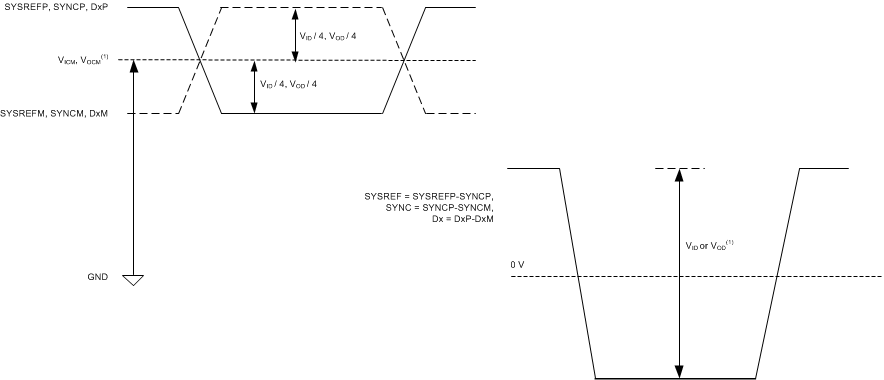VOCM is not the same as VICM. Similarly, VOD is not the same as VID.
Figure 1. Logic Levels for Digital Inputs and Outputs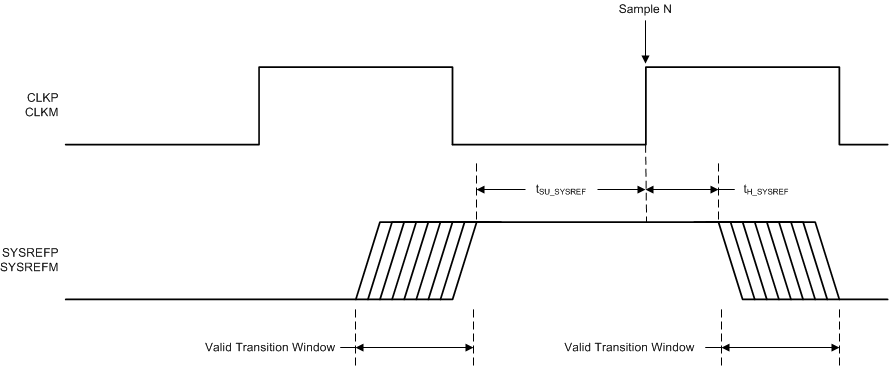Figure 2. SYSREF Timing Diagram

### 7.9 Typical Characteristics

typical values are specified at an ambient temperature of 25°C; minimum and maximum values are specified over an ambient temperature range of –40°C to +85°C; and ADC sampling rate = 1.5 GHz, 65536 points FFT, 50% clock duty cycle, AVDD19 = 1.9 V, AVDD = 1.15 V, DVDD = 1.15 V, –2-dBFS differential input, and 0-dB digital gain (unless otherwise noted)SFDR = 73 dBc, SNR = 62.4 dBFS, SINAD = 62 dBFS, THD = 71 dBc, HD2 = –75 dBFS, HD3 = –78 dBFS, SFDR (non HD2, HD3) = 85 dBc, IL spur = 81 dBFS
Figure 3. FFT for 100-MHz Input Signal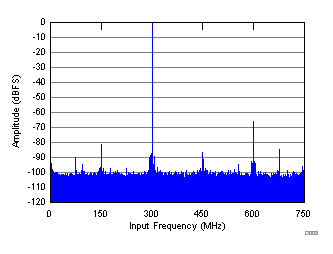SFDR = 65 dBc, SNR = 62.3 dBFS, SINAD = 61 dBFS, THD = 64 dBc, HD2 = –67 dBFS, HD3 = –75 dBFS, SFDR (non HD2, HD3) = 74 dBc, IL spur = 82 dBFS
Figure 5. FFT for 300-MHz Input Signal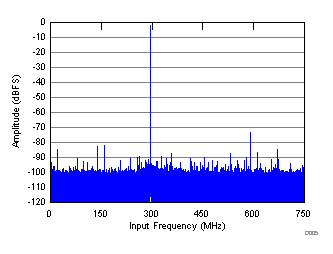SFDR = 72 dBc, SNR = 60.2 dBFS, SINAD = 60 dBFS, THD = 71 dBc, HD2 = –74 dBFS, HD3 = –87 dBFS, SFDR (non HD2, HD3) = 80 dBFS, IL spur = 80 dBFS
Figure 7. FFT for 1200-MHz Input Signal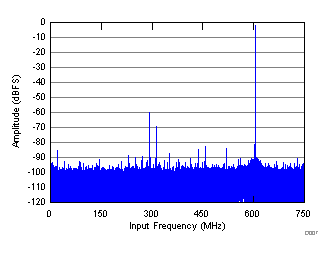SFDR = 59 dBc, SNR = 57.9 dBFS, SINAD = 56 dBFS, HD2 = –61 dBFS, HD3 = –69 dBFS, SFDR (non HD2, HD3) = 81 dBc, THD = 58 dBc, IL spur = 83 dBFS
Figure 9. FFT for 2100-MHz Input Signal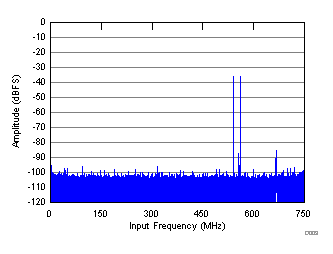fIN1 = 940 MHz, fIN2 = 960 MHz, AOUT = –36 dBFS, IMD = 94 dBFS
Figure 11. FFT for Two-Tone Input Signal (–36 dBFS)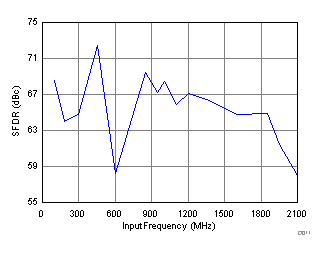Figure 13. Spurious-Free Dynamic Range vs Input FrequencyFigure 15. Signal-to-Noise Ratio vs Input Frequency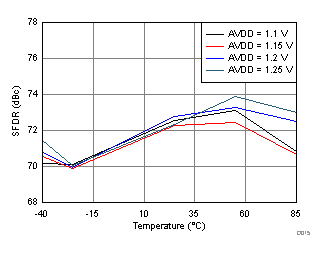fIN = 950 MHz, AIN = –2 dBFS
Figure 17. Spurious-Free Dynamic Range vs AVDD Supply and TemperaturefIN = 950 MHz, AIN = –2 dBFS
Figure 19. Spurious-Free Dynamic Range vs DVDD Supply and Temperature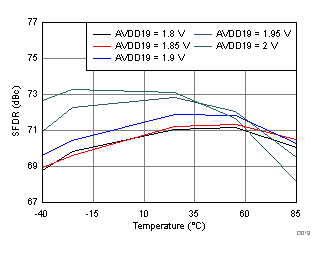fIN = 950 MHz, AIN = –2 dBFS
Figure 21. Spurious-Free Dynamic Range vs AVDD19 Supply and Temperature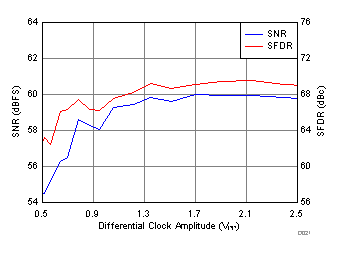fIN = 950 MHz, AIN = –2 dBFS
Figure 23. Performance vs Clock AmplitudefIN = 1850 MHz, AIN = –2 dBFS, SNR = 63.8 dBFS, SFDR (includes IL) = 78 dBc, fS = 1500 MSPS
Figure 25. FFT in 4x Decimation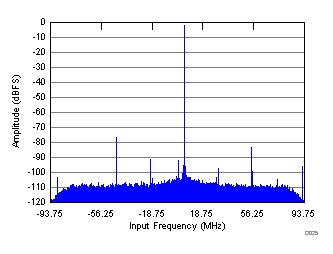fIN = 1850 MHz, AIN = –2 dBFS, SNR = 66 dBFS, SFDR (includes IL) = 77 dBc, fS = 1500 MSPS
Figure 27. FFT in 8x DecimationfIN = 1850 MHz, AIN = –2 dBFS, SNR = 65.9 dBFS, SFDR (includes IL) = 74 dBc, fS = 1500 MSPS
Figure 29. FFT in 10x Decimation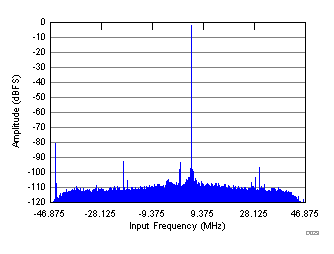fIN = 1850 MHz, AIN = –2 dBFS, SNR = 68.1 dBFS, SFDR (includes IL) = 80.9 dBc, fS = 1500 MSPS
Figure 31. FFT in 16x DecimationSFDR = 65 dBc, SNR = 62.3 dBFS, SINAD = 61 dBFS, THD = 64 dBc, HD2 = –67 dBFS, HD3 = –73 dBFS, SFDR (non HD2, HD3) = 89 dBc, IL spur = 81 dBFS
Figure 4. FFT for 185-MHz Input Signal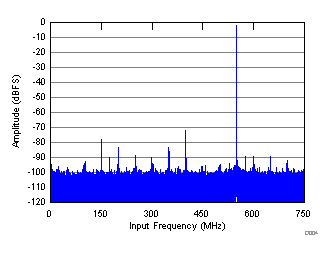SFDR = 70 dBc, SNR = 60.8 dBFS, SINAD = 60 dBFS, THD = 69 dBc, HD2 = –72 dBFS, HD3 = –78 dBFS, SFDR (non HD2, HD3) = 81 dBc, IL spur = 82 dBFS
Figure 6. FFT for 950-MHz Input Signal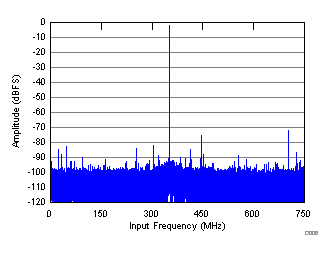SFDR = 70 dBc, SNR = 58.7 dBFS, SINAD = 58 dBFS, HD2 = –72 dBFS, HD3 = –75 dBFS, SFDR (non HD2, HD3) = 79 dBc, THD = 68 dBc, IL spur = 80 dBFS
Figure 8. FFT for 1850-MHz Input Signal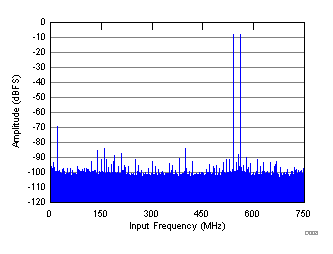fIN1 = 940 MHz, fIN2 = 960 MHz, AOUT = –8 dBFS, IMD = 75 dBFS
Figure 10. FFT for Two-Tone Input Signal (–8 dBFS)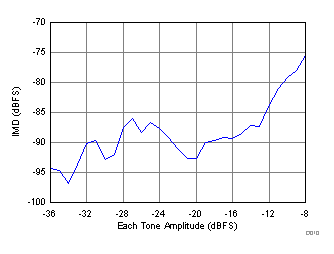Figure 12. Intermodulation Distortion vs Input Amplitude (940 MHz and 960 MHz)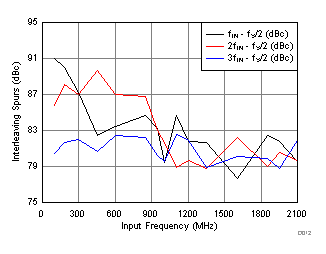Figure 14. IL Spur vs Input Frequency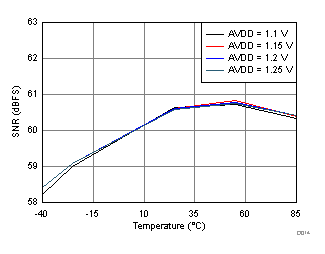fIN = 950 MHz, AIN = –2 dBFS
Figure 16. Signal-to-Noise Ratio vs AVDD Supply and Temperature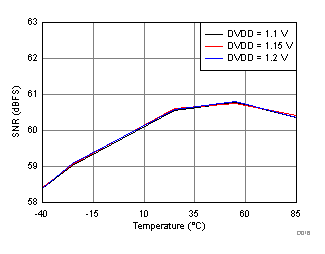fIN = 950 MHz, AIN = –2 dBFS
Figure 18. Signal-to-Noise Ratio vs DVDD Supply and Temperature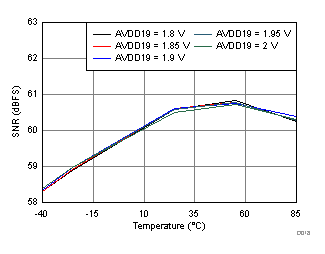fIN = 950 MHz, AIN = –2 dBFS
Figure 20. Signal-to-Noise Ratio vs AVDD19 Supply and Temperature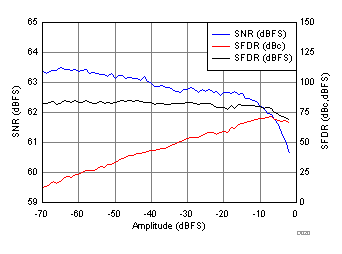fIN = 950 MHz, AIN = –2 dBFS
Figure 22. Performance vs Amplitude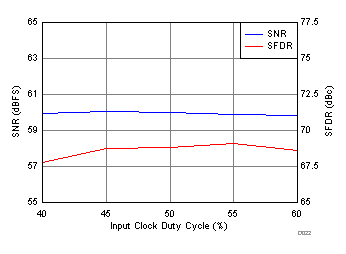fIN = 950 MHz, AIN = –2 dBFS
Figure 24. Performance vs Clock Duty Cycle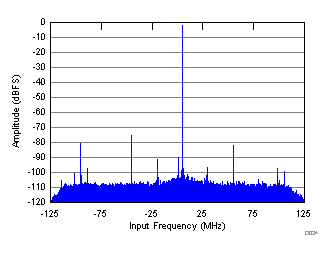fIN = 1850 MHz, AIN = –2 dBFS, SNR = 65 dBFS, SFDR (includes IL) = 75 dBc, fS = 1500 MSPS
Figure 26. FFT in 6x Decimation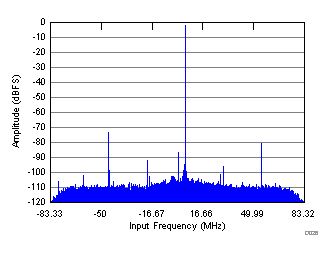fIN = 1850 MHz, AIN = –2 dBFS, SNR = 65.8 dBFS, SFDR (includes IL) = 74 dBc, fS = 1500 MSPS
Figure 28. FFT in 9x Decimation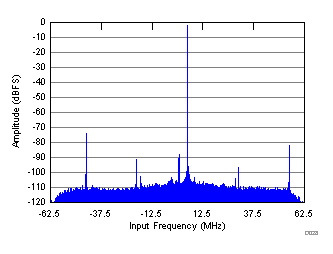fIN = 1850 MHz, AIN = –2 dBFS, SNR = 66.4 dBFS, SFDR (includes IL) = 74.1 dBc, fS = 1500 MSPS
Figure 30. FFT in 12x Decimation